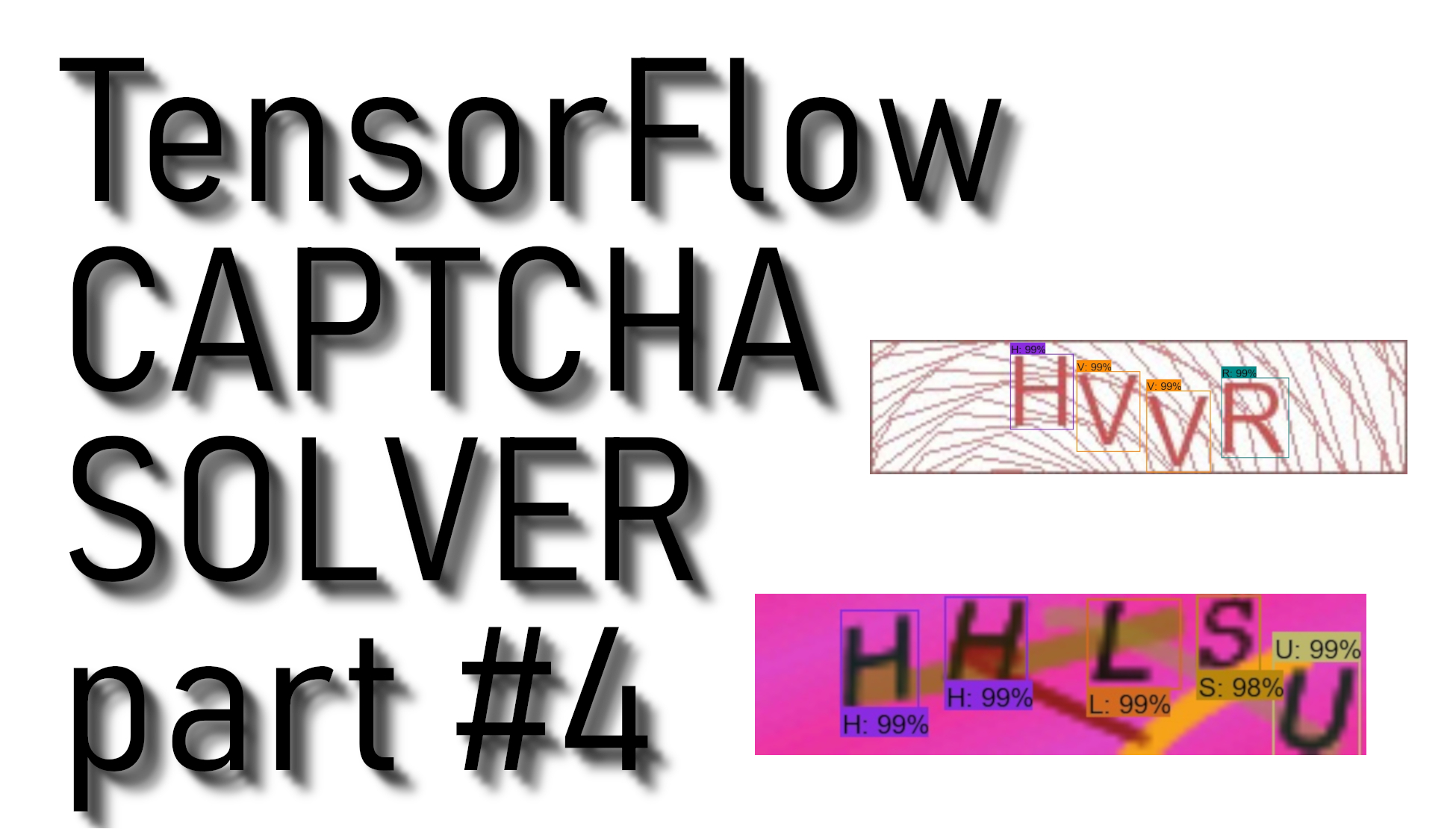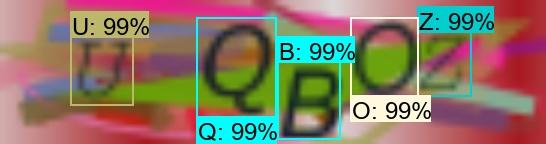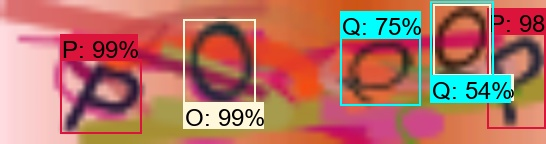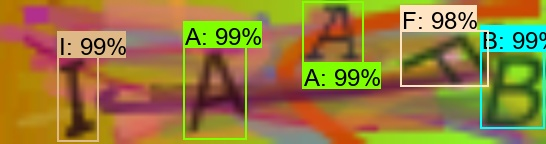# Tensorflow CAPTCHA solver final part

## In this tutorial, we will grab all CAPTCHA symbols, check the order of them, detection accuracy, and overlapping, and use these components to write the final out-of-the-box CAPTCHA solver.

In this tutorial, we continue with code from part 3, so if you didn't watch that part, I recommend doing so. In this tutorial, we only write a code to get correct CAPTCHA results from the detected image. In this tutorial, we grab all symbols from CAPTCHA, checking the order of characters, detection accuracy, and overlappings. We use all these components to write the final working out-of-the-box CAPTCHA solver.

The text version tutorial will not be as detailed as the video tutorial. If you are interested in what every line in code does and why we need that exact line, you should watch my YouTube tutorial. While writing this code, I was trying to explain every line of code. In this text version tutorial, I tried to add a few comments to the code to help you understand what that code part does.

So below is the final code, which you can copy and use. But to use it, you will need to install TensorFlow and all necessary libraries. Moreover, you will need "`CAPTCHA_frozen_inference_graph.pb`" this is my trained CAPTCHA detection model and "`CAPTCHA_labelmap.pbtxt`". When you have all your files prepared, you should be able to use this detection model. The link to download my code is above. It even includes few image examples that you could test if it works for you.

Here is the detection code:

``````# Welcome to CAPTCHA break tutorial !

# Imports
import cv2
import numpy as np
import os
import sys
# run on CPU, to run on GPU comment this line or write '0'
os.environ['CUDA_VISIBLE_DEVICES'] = '-1'
import tensorflow as tf
from distutils.version import StrictVersion
from collections import defaultdict

# title of our window

# Env setup
from object_detection.utils import ops as utils_ops
from object_detection.utils import label_map_util
from object_detection.utils import visualization_utils as vis_util

# Model preparation
# List of the strings that is used to add correct label for each box.
NUM_CLASSES = 37

# Load a (frozen) Tensorflow model into memory.
categories = label_map_util.convert_label_map_to_categories(label_map, max_num_classes=NUM_CLASSES, use_display_name=True)
category_index = label_map_util.create_category_index(categories)

detection_graph = tf.Graph()
with detection_graph.as_default():
od_graph_def = tf.GraphDef()
with tf.gfile.GFile(PATH_TO_FROZEN_GRAPH, 'rb') as fid:
od_graph_def.ParseFromString(serialized_graph)
tf.import_graph_def(od_graph_def, name='')

# Detection
with detection_graph.as_default():
with tf.Session(graph=detection_graph) as sess:
# Open image
# Resize image if needed
image_np = cv2.resize(image_np, (0,0), fx=3, fy=3)
# To get real color we do this:
image_np = cv2.cvtColor(image_np, cv2.COLOR_BGR2RGB)
# Expand dimensions since the model expects images to have shape: [1, None, None, 3]
image_np_expanded = np.expand_dims(image_np, axis=0)
# Actual detection.
image_tensor = detection_graph.get_tensor_by_name('image_tensor:0')
boxes = detection_graph.get_tensor_by_name('detection_boxes:0')
scores = detection_graph.get_tensor_by_name('detection_scores:0')
classes = detection_graph.get_tensor_by_name('detection_classes:0')
num_detections = detection_graph.get_tensor_by_name('num_detections:0')
# Visualization of the results of a detection.
(boxes, scores, classes, num_detections) = sess.run(
[boxes, scores, classes, num_detections],
feed_dict={image_tensor: image_np_expanded})
vis_util.visualize_boxes_and_labels_on_image_array(
image_np,
np.squeeze(boxes),
np.squeeze(classes).astype(np.int32),
np.squeeze(scores),
category_index,
use_normalized_coordinates=True,
line_thickness=2)
# Show image with detection
#cv2.imshow(title, cv2.cvtColor(image_np, cv2.COLOR_BGR2RGB))
# Save image with detection

# Bellow we do filtering stuff
# loop our all detection boxes
for i,b in enumerate(boxes):
for Symbol in range(37):
if classes[i] == Symbol: # check if detected class equal to our symbols
if scores[i] >= 0.65: # do something only if detected score more han 0.65
# x-left        # x-right
mid_x = (boxes[i]+boxes[i])/2 # find x coordinates center of letter
# to captcha_array array save detected Symbol, middle X coordinates and detection percentage

# rearange array acording to X coordinates datected
for number in range(20):

# Find average distance between detected symbols
average = 0
# Increase average distance error

# if average distance is larger than error distance
# check which symbol has higher detection percentage
else:

# Get final string from filtered CAPTCHA array

To use above code, we can insert "print(Captcha_detection("10.jpg"))" line or we can call this function from another file. So I created a new python script called "main_.py" and wrote following lines to it. This way we will try to solve "10.jpg" CAPTCHA:``````

To use the above code, we can insert the "print(Captcha_detection("10.jpg"))" line or we can call this function from another file. So I created a new python script called "main_.py" and wrote the following lines to it. This way we will try to solve the "10.jpg" CAPTCHA:

``````from CAPTCHA_object_detection import *

I added one line into the code to increase the size of CAPTCHAS by three times because usually, they are tiny. Without resizing the image, our model would detect it in the wrong way.

If you were trying this tutorial and worked for you, please write your experience under my YouTube video. So I will know if it worked for you or not. Also, if you tried it but it didn't work for you, you can ask me for advice under my YouTube video. I will try to help you.

Here are few CAPTCHA image results:Returned result: UQBOZReturned result: POQQPReturned result: IAAFB

That’s all for this CAPTCHA solver tutorial. I got the results that I wanted to get so that you can do the same. Now I can solve any more straightforward CAPTCHA with this code. With this code, we can't solve every difficult captcha, which has few words in it. But I think this way, we can solve at least 95% of CAPTHCA's used on the internet, except google reCAPTCHA. Thank you all for following me, and keep learning on other tutorials! In the future, I may write another tutorial to solve hard CAPTCHA with word combinations or google reCAPTCHA, but for now, I am satisfied with what I have.